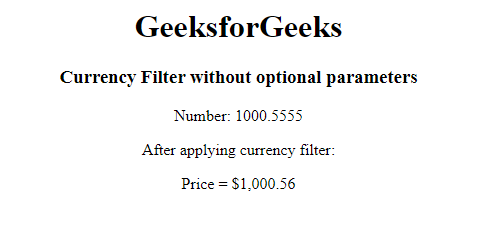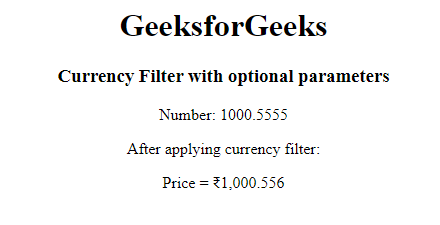Open in App
Not now

# What is currency filter in AngularJS ?

• Last Updated : 12 Apr, 2022

In this article, we will know the currency filters in AngularJS, along with understanding its implementation through the examples. Filters are used to modify or format the given data in a certain way. AngularJS has different filters such as uppercase, lowercase, etc. One of those filters is the currency filter.

The Currency filter modifies or formats a given number into a currency. In other terms, we are just taking a number and displaying it in a specific currency format.

Syntax for HTML Template:

`{{ amount | currency : symbol : fractionSize}}`

Syntax for JavaScript:

`\$filter('currency')(amount, symbol, fractionSize)`

Parameters: It accepts 2 parameters, which are described below:

• amount: The number input needed to format into currency.
• symbol: It is an optional parameter where we provide the symbol or identifier we want to display. By default, it is set to the dollar symbol.
• fractionSize: It round of the specific number to particular decimal places. It’s an optional parameter & by default, it is set to the default max for the current locale.

Example: This example describes the currency filter without using the optional parameters.

## HTML

 `<``html``> ` ` `  `<``head``> ` `    ``<``title``>Currency Filter ` `    ``<``script` `src``= ` `"https://ajax.googleapis.com/ajax/libs/angularjs/1.8.2/angular.min.js"``> ` `    `` ` ` ` ` `  `<``body``> ` `    ``<``center``> ` `        ``<``h1``>GeeksforGeeks ` `        ``<``h3``> ` `            ``Currency Filter without optional parameters ` `        `` ` `        ``<``div` `ng-app``=``"myApp"`  `             ``ng-controller``=``"currencyCtrl"``> ` `            ``<``p``>Number: {{curr}} ` `            ``<``p``>After applying currency filter: ` `            ``<``p``>Price = {{ curr | currency}} ` `        `` ` `        ``<``script``> ` `            ``var app = angular.module('myApp', []); ` `            ``var curr = 1000.5555; ` `            ``app.controller('currencyCtrl', function(\$scope) { ` `                ``\$scope.curr = curr; ` `            ``}); ` `        `` ` `    `` ` ` ` ``

Output:Currency filter without parameters

Example: This example describes the currency filter with optional parameters.

## HTML

 `<``html``> ` ` `  `<``head``> ` `    ``<``title``>Currency Filter ` `    ``<``script` `src``= ` `"https://ajax.googleapis.com/ajax/libs/angularjs/1.8.2/angular.min.js"``> ` `    `` ` ` ` ` `  `<``body``> ` `    ``<``center``> ` `        ``<``h1``>GeeksforGeeks ` `        ``<``h3``>Currency Filter with optional parameters ` `        ``<``div` `ng-app``=``"myApp"`  `             ``ng-controller``=``"currencyCtrl"``> ` `            ``<``p``>Number: {{curr}} ` `            ``<``p``>After applying currency filter: ` `            ``<``p``>Price = {{ curr | currency : "₹" :3}} ` `        `` ` `        ``<``script``> ` `            ``var app = angular.module('myApp', []); ` `            ``var curr = 1000.5555; ` `            ``app.controller('currencyCtrl', function(\$scope) { ` `                ``\$scope.curr = curr; ` `            ``}); ` `        `` ` `    `` ` ` ` ``

Output:Currency filter with parameters

My Personal Notes arrow_drop_up
Related Articles